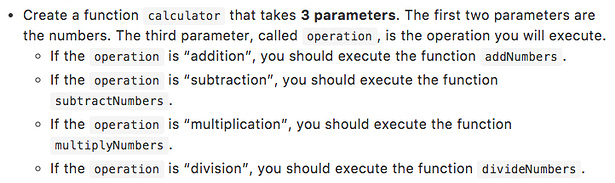# Maximum call stack size exceeded

Hey there, I’m working on a exercise and I got an unfamiliar error, and also is it my code going right? or what is it that I’m doing wrong?

You are getting the call stack error, because the calculator function is calling itself. This will keep a cycle of repeated calls until the stack overflows and this error is produced. If you want to call the calculator function, just make sure you call it outside of the calculator function’s definition.

The last part where I’m putting the “else” and the console.log the calculator it is wrong i’m guessing. But what do I have to call it otherwise to get a code. I’m doing something wrong with the exercise, what is it?

First, you have defined 3 parameters for your calculator function, but when you call it, you are only passing 2 values (6 and 10).

Instead of posting screenshots of your code, can you please post the actual code? It will make it easier for me to tinker with your code and give you back suggestions.

To enter a code block into the forum, precede it with a line of three backticks and follow it with a line of three backticks to make easier to read. See this post to find the backtick on your keyboard. The “preformatted text” tool in the editor (`</>`) will also add backticks around text.I actually did pass my code, it’s the first picture the white one

``````function addNumbers(x, y) {
return x + y;
}

function substractNumbers(b, c) {
return b - c;
}
substractNumbers(28 - 40);

function multiplyNumbers(d, f) {
return d * f;
}
multiplyNumbers(5, 25);

function divideNumbers(g, h) {
return g / h;
}

divideNumbers(100, 5);

function calculator(z, w, operation) {

if(operation === addNumbers()) {
} else if(operation === substractNumbers()) {
console.log(substractNumbers());
} else if(operation === multiplyNumbers()) {
console.log(multiplyNumbers());
} else if(operation === divideNumbers()) {
console.log(divideNumbers());
}
}

calculator(operator);
``````

The code you posted above does not meet the requirements of the instructions I see at the top (see below).I do not see where you are checking if the operation argument is equal to the string “addition”, “subtraction”, “multiplication”, or "division.

If you passed the challenge, it is only because you hardcoded the function calls it was expecting (see below).

substractNumbers(28 - 40);

multiplyNumbers(5, 25);

divideNumbers(100, 5);

I believe what the challenge was trying to get you to do was the following:

``````function addNumbers(x, y) {
return x + y;
}

function subtractNumbers(b, c) {
return b - c;
}

function multiplyNumbers(d, f) {
return d * f;
}

function divideNumbers(g, h) {
return g / h;
}

function calculator(z, w, operation) {
if (operation === 'addition') {
} else if (operation === 'subtraction') {
return subtractNumbers(z, w);
} else if (operation === 'multiplication') {
return multiplyNumbers(z, w);
} else if (operation === 'division') {
return divideNumbers(z, w);
}
}

calculator(10, 20, "addition"); // returns 30
calculator(28, 40, "subtraction"); // returns -12
calculator(5, 25, "multiplication"); // returns 125
calculator(100, 5, "division"); // returns 20
``````
1 Like

Thank you so much! You’re the best